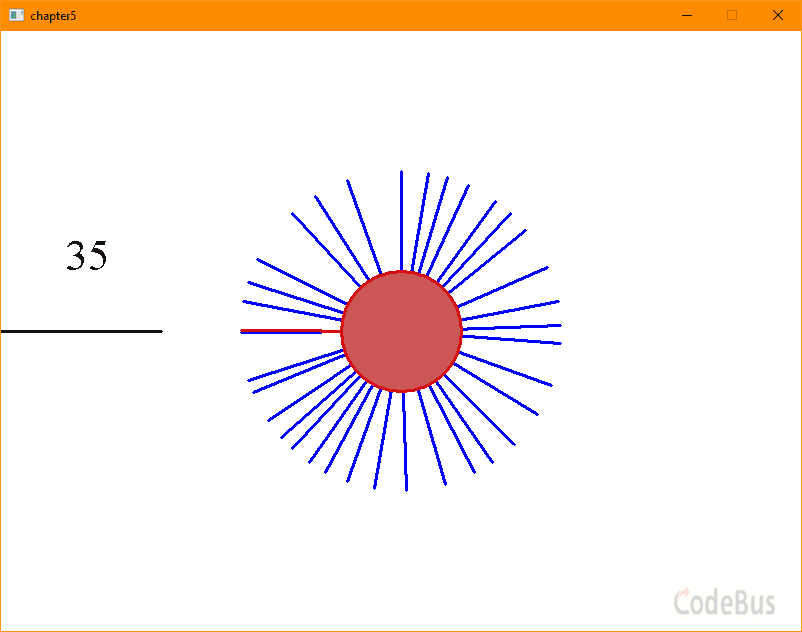# 童晶``````#include <graphics.h>
#include <conio.h>
#include <stdio.h>
#include <math.h>
int main()
{
const float Pi = 3.1415926; // Pi常量
int width = 800; // 画面宽度
int height = 600; // 画面高度
initgraph(width,height); // 新开一个画面
setbkcolor(RGB(255,255,255)); // 背景为白色
setlinestyle(PS_SOLID,3); // 线宽为3，这样针看起来更明显

float lineLength = 160; // 针的长度
float xEnd,yEnd; // 针的终点位置坐标（针起始位置为圆心）
float rotateSpeed = Pi/360; // 针的旋转速度
int lineNum = 0;  // 在旋转的针的个数
float Angles; // 浮点数数组，存储所有针的旋转角度，最多1000根针
int score = 0; // 得分
int i;

BeginBatchDraw(); // 开始批量绘制
while (1) // 重复循环
{
cleardevice(); // 以背景色清空背景
setlinecolor(RGB(0,0,0)); // 设置针颜色为黑色
line(0,height/2,lineLength,height/2); // 左边发射区域的一根针

for (i=0;i<lineNum;i++) // 对所有旋转针进行遍历
{
Angles[i] = Angles[i] + rotateSpeed; // 角度增加
if (Angles[i]>2*Pi) // 如果超过2*Pi，就减去2*Pi，防止角度数据无限增加
Angles[i] = Angles[i] - 2*Pi; //
xEnd = lineLength*cos(-Angles[i]) +width/2; // 计算针的末端坐标
yEnd = lineLength*sin(-Angles[i]) +height/2;
setlinecolor(RGB(0,0,255)); // 设定旋转针的颜色为蓝色
if (i==lineNum-1) // 最新发射的一根针，设定颜色为红色
setlinecolor(RGB(255,0,0));
line(width/2,height/2,xEnd,yEnd); // 绘制一根针
}

if (kbhit() && rotateSpeed!=0) // 如果按键，并且旋转速度不等于0
{
char input = _getch(); // 获得用户按键输入
if (input==' ') // 如果为空格键
{
lineNum++; // 针的个数加1
Angles[lineNum-1] = Pi; // 这根新增加针的初始角度
xEnd = lineLength*cos(-Angles[lineNum-1]) +width/2; //新增针的末端坐标
yEnd = lineLength*sin(-Angles[lineNum-1]) +height/2;
line(width/2,height/2,xEnd,yEnd); // 绘制出这根新增加的针
for (i=0;i<lineNum-1;i++) // 拿新增加的针和之前所有针比较
{
// 如果两根针之间角度接近，认为碰撞，游戏失败
if (abs(Angles[lineNum-1]-Angles[i]) < Pi/60)
{
rotateSpeed = 0; // 旋转速度设为0
break; // 不用再比较了，循环跳出
}
}
score = score + 1; // 得分+1
}
}
setfillcolor(HSVtoRGB(0,lineNum/60.0,0.8)); // 绘制中间的圆盘，针越多，其颜色越鲜艳
setlinecolor(HSVtoRGB(0,0.9,0.8)); // 设置圆盘线条颜色为红色
fillcircle(width/2,height/2,60); // 绘制中间的圆盘
TCHAR s; // 定义字符串数组
_stprintf(s, _T("%d"),  score); // 将score转换为字符串
settextstyle(50, 0, _T("Times")); // 设置文字大小、字体
settextcolor(RGB(50,50,50));  // 设置字体颜色
outtextxy(65, 200, s); // 输出得分文字

FlushBatchDraw(); // 批量绘制
Sleep(10); // 暂停10毫秒
}
closegraph();  // 关闭画面
return 0;
}
``````

1. 随着游戏的进行，针的旋转速度越来越快，游戏难度越来越大；

2. 中间显示为旋转蛇图案，每被针射中一次，换一次随机颜色；

3. 尝试在画面右边新增一位玩家，实现双人版的见缝插针游戏。

视频教程可以参考这里：https://zhuanlan.zhihu.com/p/195049797

### 评论 (3) -

•请问这个程序的时间复杂度和空间复杂度是多少？
•在VS2019环境中，要改3处才能正常编译运行
kbhit改_kbhit
abs改fabs
_stprintf改swprintf_s
•谢谢两位大佬，
成功运行了